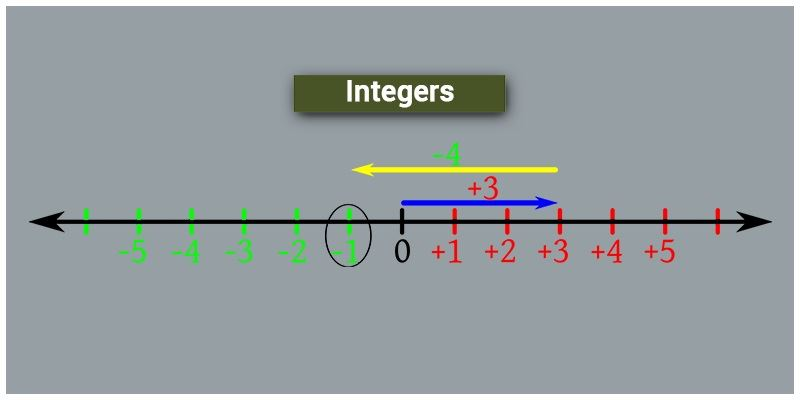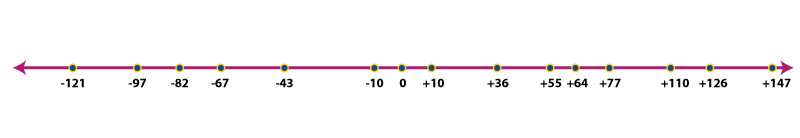# Integers

In Maths, integers are the numbers which can be positive, negative or zero. These numbers are used to perform various arithmetic calculations, like addition, subtraction, multiplication and division. The examples of integers are, 1, 2, 5,8, -9, -12, etc. We have different types of numbers in Mathematics, such as;

• Real Numbers
• Natural Numbers
• Whole Numbers
• Even and Odd Numbers, etc.

But here we are going to discuss about integers, its definition and types.

## What are Math Integers?

The word integer originated from the Latin word “Integer” which means whole. It is a special set of whole numbers comprised of zero, positive numbers and negative numbers and denoted by the letter Z.
Examples of Integers – 1, 6, 15.
Fractions, decimals, and percents are out of this basket. Examples – -2.4, 3/4, 90.6.

There are three Properties of Integers:

1. Associative
2. Commutative
3. Distributive

We are living in a world of numbers where everything depends on numbers. Yet they are expressed or represented in different forms and share few general characteristics. In order to differentiate each of them, mathematicians initiated the grouping system. They categorized numbers based on their similarities and dissimilarities. These gave birth to the types of numbers like natural numbers, whole numbers, rational numbers, real and imaginary numbers and so on.Example: -100,-12,-1, 0, 2, 1000, 989 etc…

As a set, it can be represented as follows:

Z= {……-8,-7,-6, -5, -4, -3, -2, -1, 0, 1, 2, 3, 4, 5,6,7,8,……}

## Types of Integers

Integers comes in three types:

• Zero
• Postive Numbers(Natural) and
• Negative Numbers(Negatives of Natural Numbers).

### Positive numbers

Positive numbers are those numbers which are having a plus sign (+). Most of the time positive numbers are represented simply as a whole number without the plus sign (+). Every positive number is greater than zero as well as negative numbers. On a number line, positive numbers are represented to the right of zero.

Example: 1,2, 200, 5666,99999999, etc.

### Negative numbers

In contrast to positive numbers, negative numbers are numbers symbolized with a minus sign (-). Negative numbers are represented to the left of zero on a number line.

Example: …., -99999, -150, -10, -1.

### Zero

Zero is neither a positive nor a negative integer. It is a neutral number i.e. zero has no sign (+ or -).

Learn how to add and subtract integers here.

## Applications Of Integers

Integers are not just numbers on a paper; they have many real life applications. Effect of positive and negative numbers in the real world is different. They are mainly used to symbolize two contradicting situations.

For example, when the temperature is above zero, positive numbers are used to denote temperature whereas negative numbers denote the temperature below zero. They help one to compare and measure two things like how big or small or more or fewer things are and hence can quantify things.

Some real life situations where integers come into play are player’s scores in golf, football and hockey tournaments, the rating of movies or songs, in banks credits and debits are represented as positive and negative amounts respectively.

### Sample Problem:

Question : Plot the following integers on the number line: -121, -97, -82, -67, -43, -10, 0, 10, 36, 55, 64, 77, 110, 126, 147.